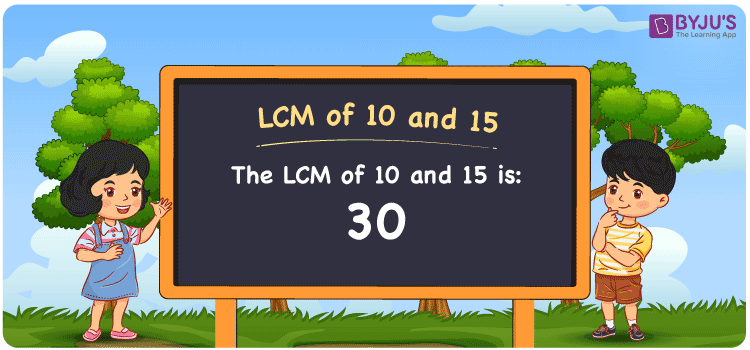Checkout JEE MAINS 2022 Question Paper Analysis : Checkout JEE MAINS 2022 Question Paper Analysis :

# LCM of 10 and 15

LCM of 10 and 15 is 30. The LCM of any two numbers is the number evenly divisible by the two given numbers. Least common multiple of 10 and 15 is the value we get from the common multiples. (10, 20, 30, 40, 50, 60, 70, ….) and (15, 30, 45, 60, 75, 90,….) are the multiples of 10 and 15. We can find the LCM of two numbers using methods such as prime factorisation, division and by listing the multiples.

## What is LCM of 10 and 15?

The answer to this question is 30. The LCM of 10 and 15 using various methods is shown in this article for your reference. The LCM of two non-zero integers, 10 and 15, is the smallest positive integer 30 which is divisible by both 10 and 15 with no remainder.## How to Find LCM of 10 and 15?

LCM of 10 and 15 can be found using three methods:

• Prime Factorisation
• Division method
• Listing the multiples

### LCM of 10 and 15 Using Prime Factorisation Method

The prime factorisation of 10 and 15, respectively, is given by:

10 = 2 x 5 = 2¹ x 5¹

15 = 3 x 5 = 3¹ x 5¹

LCM (10, 15) = 30

### LCM of 10 and 15 Using Division Method

We’ll divide the numbers (10, 15) by their prime factors to get the LCM of 10 and 15 using the division method (preferably common). The LCM of 10 and 15 is calculated by multiplying these divisors.

 2 10 15 3 5 15 5 5 5 1 1

No further division can be done.

Hence, LCM (10, 15) = 30

### LCM of 10 and 15 Using Listing the Multiples

To calculate the LCM of 10 and 15 by listing out the common multiples, list the multiples as shown below

 Multiples of 10 Multiples of 15 10 15 20 30 30 45 40 60 50 75

LCM (10, 15) = 30

## Video Lesson on Applications of LCM## LCM of 10 and 15 Solved Examples

Determine the smallest number which is exactly divisible by 10 and 15.

Solution:

We know that

The smallest number which is exactly divisible by 10 and 15 is the LCM.

Multiples of 10 = 10, 20, 30, 40, 50, 60, 70, …..

Multiples of 15 = 15, 30, 45, 60, 75, 90, 105, ….

Hence, the LCM of 10 and 15 is 30.

## Frequently Asked Questions on LCM of 10 and 15

### Find the LCM of 10 and 15 using the prime factorization method.

To determine the LCM using the prime factorization, we first have to find the prime factors.

10 = 2 x 5

15 = 3 x 5

LCM of 10 and 15 is the product of prime factors raised to the highest exponent among the numbers 10 and 15

LCM of 10 and 15 = 2¹ x 3¹ x 5¹ = 30

### What is the GCF if the LCM of 10 and 15 is 30?

LCM x GCF = 10 x 15

Given

LCM of 10 and 15 = 30

30 x GCF = 150

GCF = 150/30 = 5

### Write the relation between GCF and LCM of 10 and 15.

The relation between GCF and LCM of 10 and 15 is

LCM x GCF = 10 x 15

LCM x GCF = 150